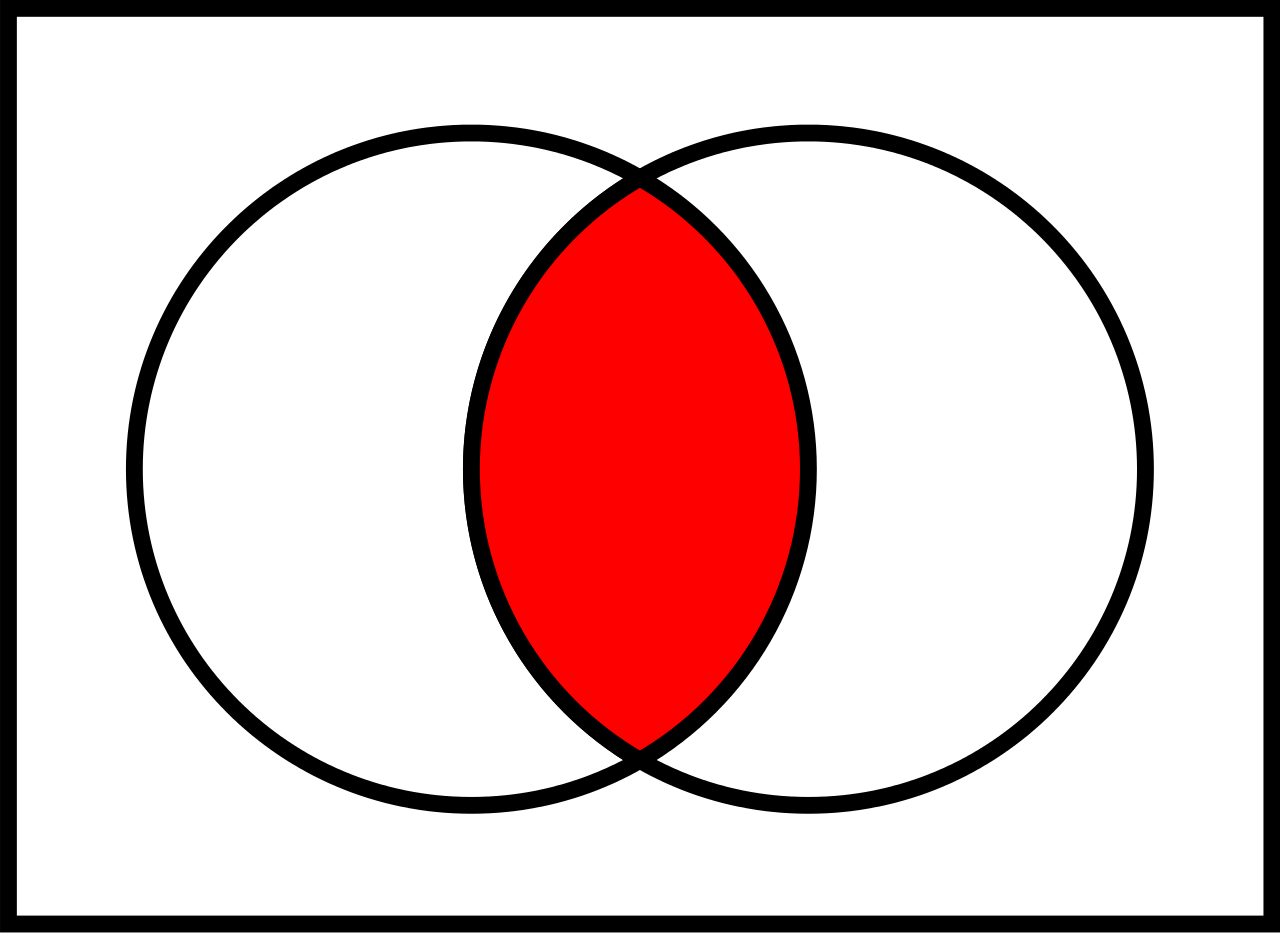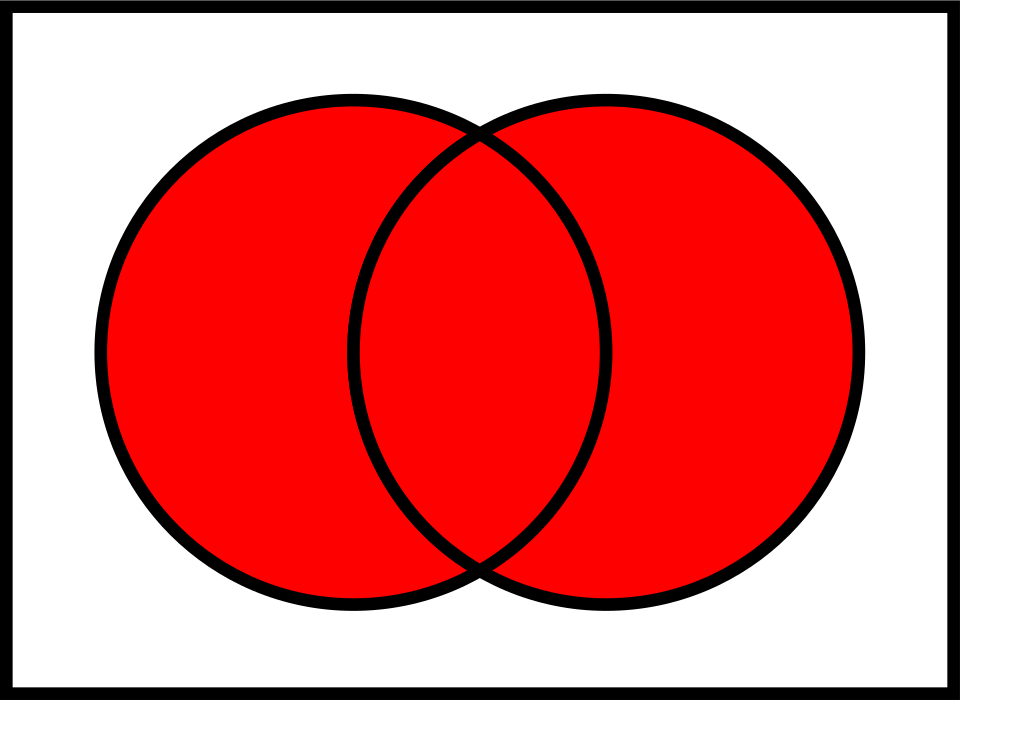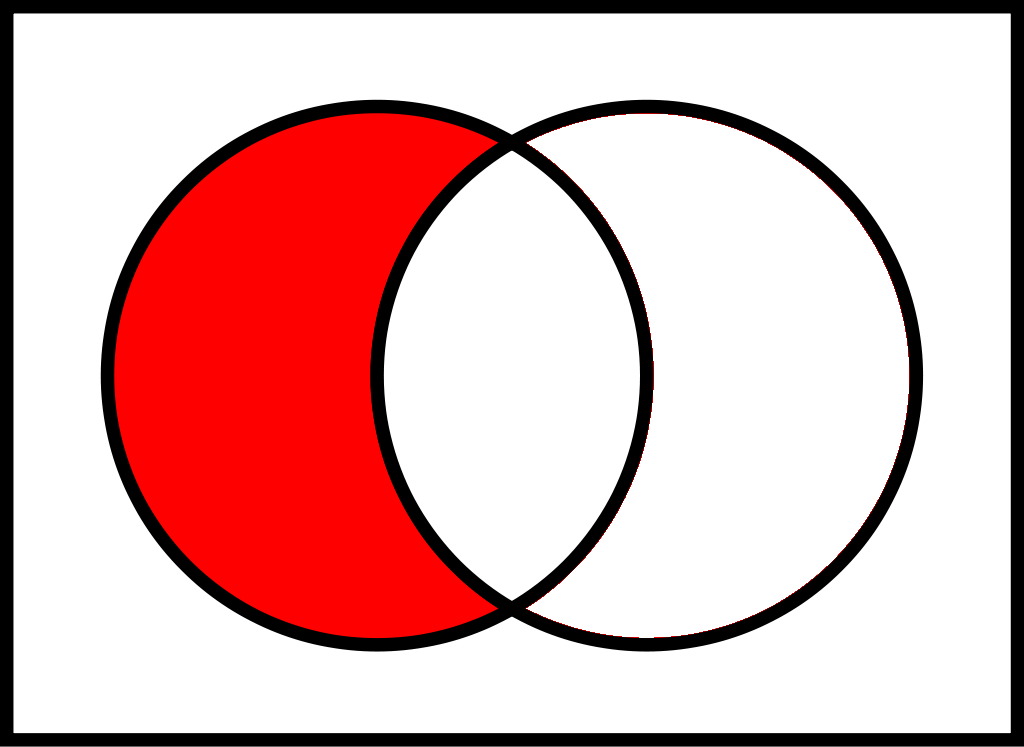## Introduction

Both the Series and DataFrame objects contain an explicit index that alows us to reference the data. It can be thought of either as an immutable array or as an indexed set but with (possibly) repeated values. In fact, an index object is indexed and ordered but immutable.

We can create an index object explicitly using the constructor function pd.Index().

Example

Creating an index object.

1pd.Index([1,2,3,4])

Int64Index([1, 2, 3, 4], dtype='int64')


Repetitions are allowed.

Example

Creating an index object with repeated indices.

1pd.Index([1, 2, 3, 2, 1, 4])

Int64Index([1, 2, 3, 2, 1, 4], dtype='int64')


## Index as an Immutable Array

Example

Creating a random index object.

1ix = pd.Index(rd.choices(range(1,4),k=6))
2ix

Int64Index([2, 3, 2, 3, 3, 3], dtype='int64')


We can use Python indexing notation to retrieve values or perform slicing.

Example

Access values of an index object using indexing.

1ix

3


Example

Access values of an index object using slicing.

1ix[2:5]

Int64Index([2, 3, 3], dtype='int64')


However, an index object is immutable.

Example

Index object is immutable.

1ix=88

TypeError                                 Traceback (most recent call last)

Cell In , line 1
----> 1 ix=88

-> 5021     raise TypeError("Index does not support mutable operations")

TypeError: Index does not support mutable operations


## Index as an Ordered Set

The Index object follows many of the conventions used by Python’s built-in set data structure, so that unions, intersections, differences, and other combinations can be computed in a familiar way.

Let’s first create two index objects.

Example

Creating two index objects.

1ix1 = pd.Index(rd.sample(range(1,10),k=6))
2print(ix1)
3ix2 = pd.Index(rd.sample(range(1,7),k=6))
4print(ix2)

Int64Index([8, 5, 2, 3, 9, 7], dtype='int64')
Int64Index([5, 1, 6, 2, 3, 4], dtype='int64')


The intersection of two sets $A$ and $B$, denoted as $A \cap B$, is the set of all elements that are in both $A$ and $B$.Example

Intersection of two index objects.

1ix1.intersection(ix2)

Int64Index([5, 2, 3], dtype='int64')


The union of two sets $A$ and $B$, denoted as $A \cup B$, is the set of all elements that are in either $A$ or $B$ (or both).Example

Union of two index objects.

1ix1.union(ix2)

Int64Index([1, 2, 3, 4, 5, 6, 7, 8, 9], dtype='int64')


The difference of two sets $A$ and $B$, denoted as $(A-B)$, is the set of all elements of $A$ that are not in $B$.Example

Difference of two index objects.

1ix1.difference(ix2)

Int64Index([7, 8, 9], dtype='int64')


The above results in the set (ix1-ix2), that is, the set of all elements in ix1 that are not in ix2.

The symmetric difference of two sets $A$ and $B$, given as $A\ominus B$, can be computed as $(A^c \cap B) \cup (B^c \cap A)$ or as $(A \cup B) - (A \cap B)$.Example

Symmetric difference of two index objects.

1ix1.symmetric_difference(ix2)

Int64Index([1, 4, 6, 7, 8, 9], dtype='int64')